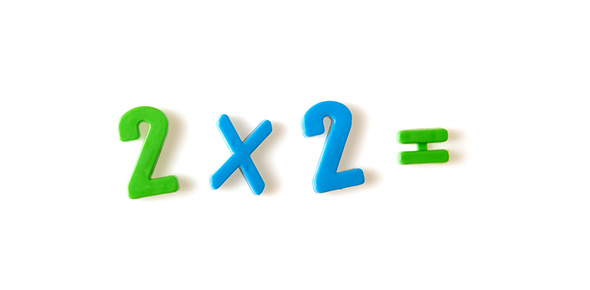# Timed Multiplication 12x12

50 Questions | Total Attempts: 85SettingsTest your memory of the 12x12 multiplication table with this timed multiplication speed test. You have three minutes to answer 50 multiplication questions. The questions are shuffled each time you take the exam. Try to be both fast and accurate! At the end of the exam, you receive a grade based on the number of questions you answered correctly. You can also review the questions you missed. Grading Scale:93%-100% = A86%-92% = B78%-85% = C70%-77% = DBelow 70% = FNeed to review? Print out this free printable multiplication chart.

• 1.
5 x 4 =
• 2.
9 x 9 =
• 3.
12 x 3 =
• 4.
7 x 4 =
• 5.
9 x 6 =
• 6.
8 x 5 =
• 7.
9 x 12 =
• 8.
11 x 5 =
• 9.
2 x 3 =
• 10.
4 x 10 =
• 11.
8 x 9 =
• 12.
12 x 11 =
• 13.
7 x 3 =
• 14.
4 x 3 =
• 15.
8 x 4 =
• 16.
5 x 7 =
• 17.
9 x 4 =
• 18.
12 x 9 =
• 19.
10 x 8 =
• 20.
9 x 7 =
• 21.
6 x 6 =
• 22.
0 x 0 =
• 23.
8 x 7 =
• 24.
5 x 5 =
• 25.
9 x 5 =
Related Topics